# Configure a Calculated Channel With a Formula (General Steps)

You may have created a new calculated channel using the Manual Site Creation tool, or via F.A.C.E.: Configure Sites. Now, you must configure the channel with a F.A.C.E. calculation, that will be applied to all the data that is received by this channel. This topic provides general directions for configuring your calculated channel with a F.A.C.E. formula.

The formula option in FACE is a popular choice because it enables you to manually define an equation using basic math, trigonometric formulas, the Circle equation and IF/ELSE statements; and apply the equation to each individual row of data from the source channel.

This article assumes that you have already created a calculated channel and are now ready to setup the channel's calculation using F.A.C.E. If you don't already have a calculated channel established, you will need to first create one before proceeding further in this topic. You can make a new calculated channel in two ways:
1) Use Manual Site Creation to add a new site that has one or more calculated channels;
2) Add a calculated channel to an existing site via F.A.C.E.: Configure Sites page.
See the Add a raw or calculated data channel to an existing site documentation.

Before continuing on in this topic, please review the following articles.

The User Guide also discusses some particular scenarios for using formulas and functions. See the following topics for details, including step-by-step instructions for replicating the use-case.

## 1. Open Configure Sites (FACE)

1. Click on Manage. A drop-down menu opens.
2. Select Configure Sites (FACE)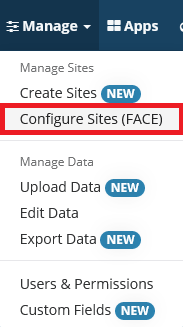## 2. View the Calculated Channel

1. Use the Choose Site dropdown list to select the site that contains the calculated channel.
2. Use the Choose Calculated Channel dropdown list to select the calculated channel you want to configure.
3. Click View Calculated Channel.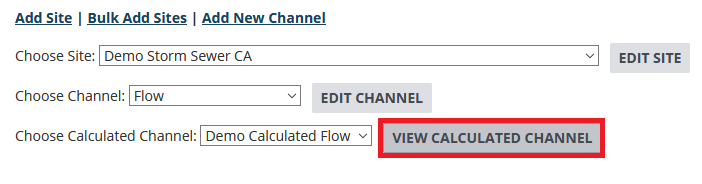## 3. Click Create Calculation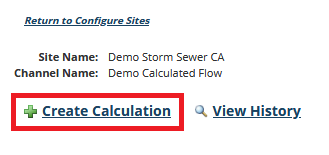## 4. Add a Calculation Name and Description, and Choose Formula

1. (Optional) Provide a Calculation Name and Description.
2. Using the Type dropdown, select the calculation you wish to use.
For this example, choose Formula to manually input a mathematical equation. Upon choosing this option, the bottom section of the screen will update to reveal further setup options.
3. (Optional) Set a Start Date and End Date.
This will apply the calculation only to data that falls within the given date range.

The Calculation Type called Circle is not present in the Type list because it is used as part of a formula. To use Circle, select Formula, and incorporate CIRCLE into your equation appropriately.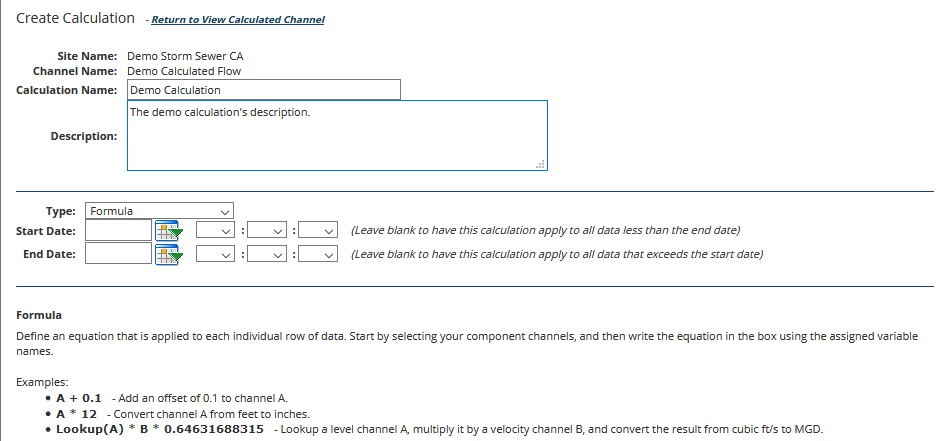## 5. Select Your Component Channels

You must specify one or more source components. For each, select the source Site and Channel, then give it a unique Variable Name (any letter).

Use + to add more source components.

NOTE: The source component you define are important for your calculated channel data output. If you are defining a constant value in your equation (ex: -0.104), the source component being applied in your calculated channel needs to have the same time-series intervals, otherwise a value will not be output. If multiple source components are defined in a channel, delete any components not being used in the equation as this may create unwanted dependencies.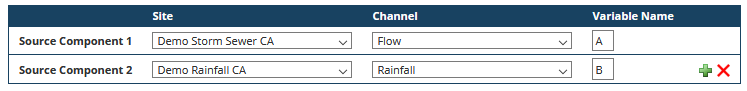## 6. (Optional) Choose a Lookup Table

This option enables you to use a lookup table in a formula. Choose a desired Lookup Table from the drop-down list and then reference it in the formula along with an input channel. For example, you could write "LOOKUP (A)".## 7. Define the Equation

You can use basic math syntax, trigonometric formulas, the Circle equation and/or IF/ELSE statements, referencing the variables with the names you previously assigned where appropriate. In this example, the CIRCLE function is used.## 8. (Optional) Configure Advanced Options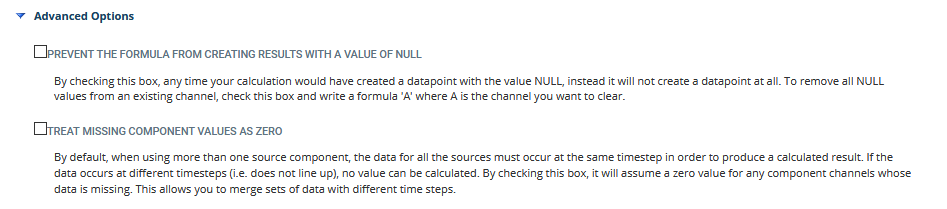• Prevent the formula from creating results with a value of NULL
Any time your calculation creates a data point with the value NULL, the addition of this point in the data set will be omitted. To remove NULL values from an existing channel, check this box an write a formula 'A', where A is the channel you want to clear.
• Treat missing component values as zero
When you are using more than one source component, by default the data for all the sources must occur at the same time (by having the same timestamp) to produce a calculated result. If the data occurs at different timestamps (data doesn't line up), no value can be calculated. By checking this box, FACE will assume a zero value for any component channels that has missing data. This allows you to merge sets of data with different timestamps.

## 9. (Optional) Add this Calculation to Other SitesThis list only contains sites that have a calculated channel with the same name, and all of the required source component channels. Take caution when applying this option to non-identical calculations to prevent unintended and inaccurate calculation results.

## 10. Click Save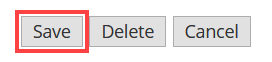FlowWorks will immediately apply the calculation to the data to create the new calculated channel. You will be taken back to the View Calculated Channels page, where the new calculation will appear in a list.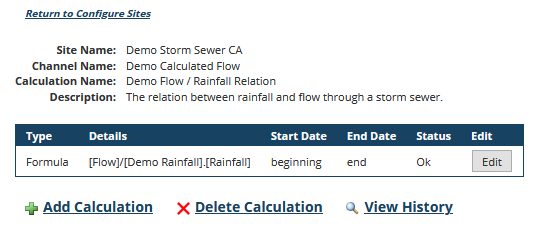Your new calculated channel is now ready for use. All incoming data from the source channels will be copied into this calculated channel, in which the conversion formula will be automatically applied to generate virtual (calculated) data. you can use this channel in the same ways as you can use raw channels, in any of FlowWorks' analysis, reporting and management tools.